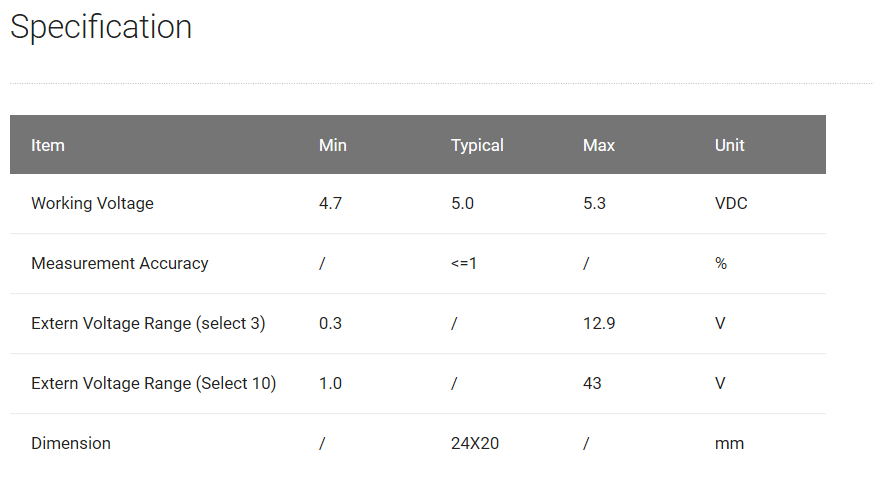# Voltaje divider supply

Hello,

I’m building an outdoor sensor supplied by a LiPo battery and a solar panel. In order to know the charge of the battery I’m planning to use the Grove Voltaje Divider. My question is: can I supply the Grove Voltaje Divider with de 3.3-3.6v the battery supplies? In case it’s not possible, how can I measure the charge of the battery in such conditions?

Thanks.

Hi there ~

The range of working Voltage of Grove Voltage Divider is from 4.7 to 5.3v, so you can not use the battery.

This is the specification of the voltage divider.Thanks cheers

JL

Ok.

So, does anybody know where can I get another voltage divider designed to work with the voltage supplied by the battery used in my design (a LiPo battery at 3.7V)?

Hello

Are you using Arduino board to build your sensor? If you’re using it, normally the working voltage of Arduino is 5V, and you can connect your battery directly to analog port (The max output voltage of Lipo battery is 3.7V), and if your working voltage of Arduino is 3.3V, you can use two 1k resistances connected in series to get the voltage divided.

By the way, if there is an ADC module in your design, in theory you can use it to get the charge of the battery and you don’t need a another voltage divider. If you have any other questions, give your feedback to us.Thanks sincerely,

JL